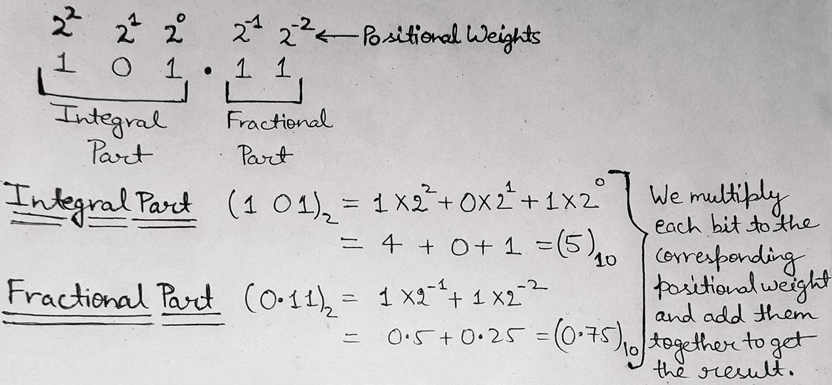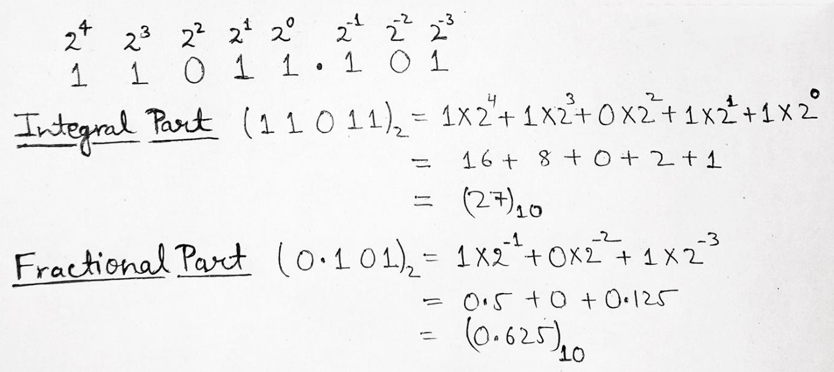# Binary to Decimal Number System Conversion

In this tutorial, we will learn about the conversion of binary to decimal number systems with the help of examples. By Saurabh Gupta Last updated : May 10, 2023

Prerequisite: Number systems

## Binary to Decimal Number System Conversion

To convert binary number to its respective decimal number we use the place values or what we call the positional weights and multiply it with the corresponding bit and add them all together to obtain the decimal number.

• In an integral part of the binary number, the weights follow the pattern as 20, 21, 22, 23, 24, 25 and so on from right to left.
• In the fractional part of the binary number, the weights follow the pattern as 2-1, 2-2, 2-3, 2-4, 2-5 and so on from left to right.

To convert a mixed binary number, we convert its integral and fractional part individually and then combine them to get the desired decimal number.

Let's take a few examples to understand the concept better.

### Example 1: Convert (101.11)2 to ( ? )10

#### SolutionNow, after converting both integral part and fractional part separately, we combine them and get the desired decimal number for the binary number i.e., (101.11)2 = (5.75)10

### Example 2: Convert (11011.101)2 to ( ? )10

#### SolutionNow, after converting both integral part and fractional part separately, we combine them and get the desired decimal number for the binary number i.e., (11011.101)2 = (27.625)10